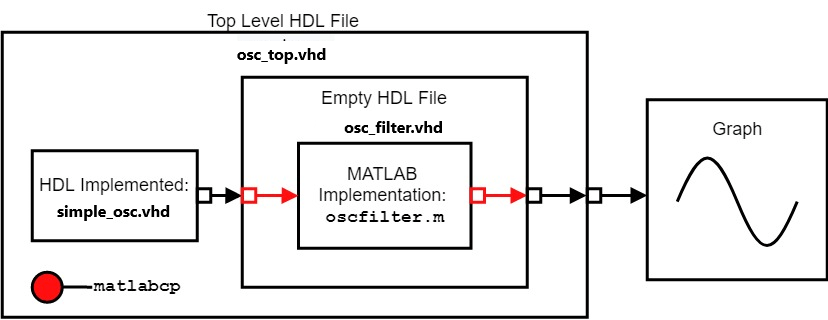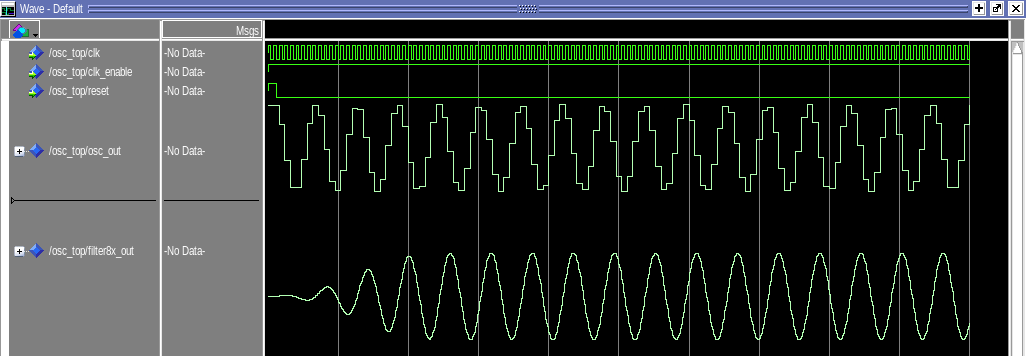# Implement Filter Component of Oscillator in MATLAB

This example shows how to implement a filter component for an HDL project in Mentor Graphics® ModelSim® and Questa® or Cadence® Xcelium™ by using the HDL Verifier™ function `matlabcp`. Instead of using HDL to model a complex component for use in an HDL project, you can use `matlabcp` to implement the component instead.

In this example, the project compiles an oscillator in VHDL® and defines the filter component using MATLAB®, before running an HDL simulation. The oscillator is a simple fixed-point sine wave generator written in HDL, and feeds a low-pass filter. This low-pass filter is a 255th order (256-tap) filter with 8x oversampling that is implemented using the polyphase technique in MATLAB. The filter inputs and outputs are carried by `matlabcp` between the HDL simulator and MATLAB, with the results displayed using the simulator waveform viewer. By using simulator waveform viewer to display the results, this example demonstrates that `matlabcp` can seamlessly integrate a component designed in MATLAB into a larger HDL workflow without requiring MATLAB in the later steps. This diagram shows the overall flow of this cosimulation process.This example considers the point of view of an HDL developer, so the main emphasis of this example will be on using the HDL simulator and command-line terminal to perform testing, and using MATLAB to implement some of the project components.

### Overview of Design Files and Script Files

This example uses two types of files: design files and script files.

Design Files

• The file `simple_osc.vhd` contains the simple sinusoidal oscillator designed using VHDL.

• The file `osc_filter.vhd` is the empty component that is used for `matlabcp` to receive the component inputs and transmit the component outputs.

• The file `osc_top.vhd` contains the top-level model of the oscillator and low-pass filter.

• The file `oscfilter.m` contains the behavioral implementation of the filter, implemented in MATLAB.

Script Files

• The file `qcommands_osc.tcl` contains the TCL commands that are sent into ModelSim/Questasim for cosimulation to occur.

• The file `tclcmd_cosim_xc.m` contains TCL commands that are sent into Xcelium for cosimulation to occur.

### Bind MATLAB Function with HDL Module

You can bind a MATLAB function with an HDL module using `matlabcp`. You need an empty HDL component with input and output ports declared. These files are necessary as `matlabcp` uses the empty component as a shell for communication between MATLAB and the HDL simulator, while the MATLAB function provides the functionality. Using `matlabcp` when verifying a single component allows MATLAB to simulate the various parts of the system, saving time and effort needed to create HDL code for testing. This approach shifts the focus onto generating tests and testing the component and creating a more efficient verification workflow. For information on creating the necessary files for `matlabcp`, see Create a MATLAB Component Function.

### Run Cosimulation

This example requires access to ModelSim/Questasim or Xcelium and MATLAB from the terminal. You must include these simulators on the system path. To start cosimulation, open MATLAB and run the `hdldaemon` command for communication between the HDL simulator and MATLAB. To complete these actions, use these commands based on operating system.

• Windows: `matlab -nodesktop -r "hdldaemon"`

• Linux: `xterm -e "matlab -nodesktop -r "hdldaemon"" &`

This opens MATLAB in a separate terminal window, in headless mode, and starts the HDL Link MATLAB server. The terminal that is running MATLAB displays this message.

`"HDLDaemon shared memory server is running with 0 connections"`

After this message appears, start the cosimulation by entering the applicable command (based on the simulator and operating system) into MATLAB command window.

• Windows and Linux:

```To launch ModelSim/Questasim and execute TCL commands in the simulator, run this command.```
```vsim('tclstart','do qcommands_osc.tcl'); ```

Xcelium

1. Run the MATLAB script `tclcmd_osc_xc.m` by entering this command at the MATLAB command prompt.

```tclcmd_osc_xc; ```

2. Launch Xcelium by entering this command at the MATLAB command prompt.

```nclaunch('tclstart', tclcmds); ```

After you enter the respective commands, cosimulation starts and produces these plots in the respective HDL simulator. Your plots might appear different right after cosimulation, but zooming to encompass the entire time axis can yield similar looking plots.

ModelSim/QuestasimXcelium### Summary

This example shows how to use MATLAB as a substitute for a major component in the HDL project, replacing the HDL implementation of the low-pass filter. The file `osc_filter.vhd` is a placeholder in the system to feed the inputs into the MATLAB function and send the outputs from the MATLAB function into the rest of the project. The implementation of the filter and process of filtering the input to produce the output is completely driven by the MATLAB function.

You can use MATLAB to replace various components in the HDL simulation project with behavioral implementations in MATLAB, less time is needed to prepare the HDL project. This approach shifts the focus onto testing and verification of the component in question, instead of spending time verifying and creating the other components of the project.